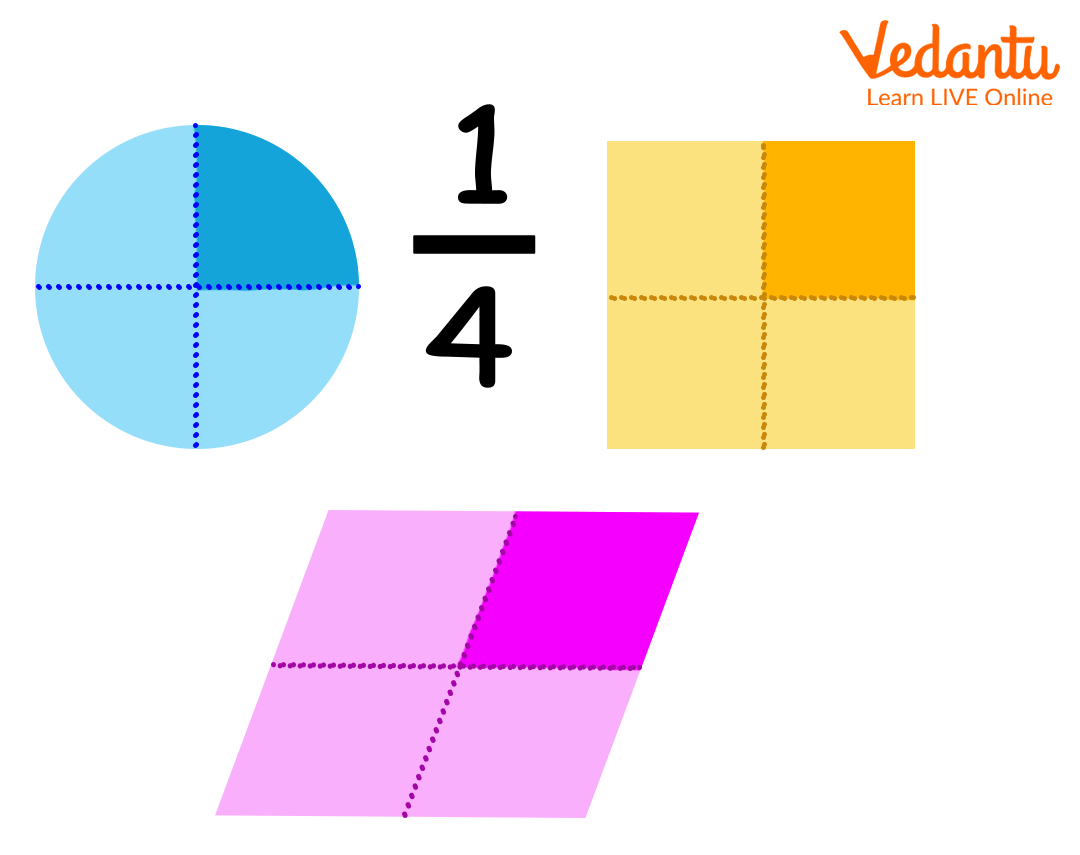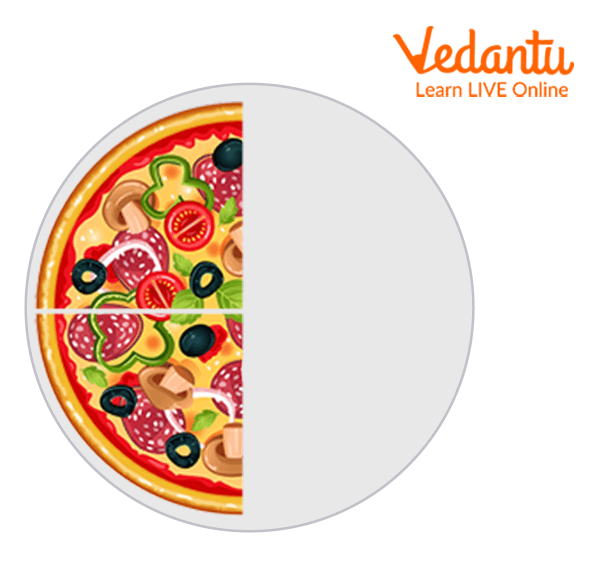Courses
Courses for Kids
Free study material
Free LIVE classes
More

# Quarter in Fraction for Kids## What is a Fraction?

Let’s first know what fraction is before coming into quarters in fraction.

A fraction is nothing but a part of something. For example, count the whole things like the pies given below.Image: 4 pizzas

Above, you can see four whole pizzas. But, what if we take away one part of the pizza as shown below.Image: One-fourth of a pizza

Here, the pizza has been cut into four equal parts. One part has been taken away. Each slice of a pizza is a part of a whole pie. So, each part is some fraction of the whole pizza.

This implies that a fraction is a part of something. Read ahead to know more about fractions, a quarter in fractions and how we can use fractions in daily life.

## How to Write Fractions?

A fraction consists of two numbers separated by a horizontal line, also known as fraction bar. The number above the horizontal line is known as the numerator. It represents the number of parts we have. The number below the horizontal line is known as the denominator. It represents the number of parts the whole is divided into.

For Example:

Look at the pizza given below:Image: One-fourth pizza

Here, the fraction is written as: 1 4

Here, the numerator 1 says that one slice of pizza is left.

And the denominator 4 says the pizza is cut into 4 equal slices.

We say this fraction is a quarter or one - quarter.

## What are Quarters in Fraction?

The quarter in fraction is one out of 4 equal parts. It is written as 1 4.

Look at the image below to understand it better.Images: Quarters in fraction

Here, this circle, square, and rectangle has been cut into quarters, and one quarter in each shape is darker.

## What is One Quarter?

One quarter means one of four equal parts.

For example, there are four members in a family. You divide the pizza into 4 equal parts and each member gets an equal share. Hence, when a whole is divided into 4 equal parts, each part is known as one quarter.Image: One quarter

It is written as 14 and read as one quarter or one-fourth.

## What are Two Quarters?

Two quarters mean two of four equal parts.

Suppose, you divide the pizza into 4 equal parts and two slices of pizza are left.Image: Two Quarters

It is written as 24 and read as two quarters or two-fourth.

## What are Three Quarters?

Three quarters means three of four equal parts. It is written as 34 read as three quarters or three- fourth.

Suppose, you divide the pizza into 4 equal parts and three pieces of pizza are left.Image: Three quarters

## Conclusion

In conclusion, a quarter is one of four equal parts into which something is divided. Also, two quarters means two of four equal parts and three quarters means three of four equal parts. If you enjoyed studying fractions and one quarter, two quarters and three quarters, and would love to read similar topics, visit us on our website.

Last updated date: 26th Sep 2023
Total views: 137.4k
Views today: 3.37k

## FAQs on Quarter in Fraction for Kids

1. How many quarters make a whole?

A whole is made up of four quarters. This implies that one whole is divided into four equal parts.

2. How many quarters are there in 3 whole pizzas?

There are 12 quarters in 3 whole pizzas. This is because we know quarters means one of four equal parts. Accordingly, if one pizza has 4 quarters then eventually 3 pizzas will have 12 quarters ( 4 3 = 12).

3. How do you write three quarters in a fraction?

Three quarters in a fraction is written as 3/4. This means 3 parts out of 4. Here, the top number ‘3’ is known as numerator and the bottom number ‘4’ is known as denominator.